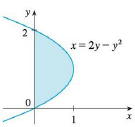Chapter 5.4, Problem 49E### Single Variable Calculus: Early Tr...

8th Edition
James Stewart
ISBN: 9781305270343

#### Solutions

Chapter
Section### Single Variable Calculus: Early Tr...

8th Edition
James Stewart
ISBN: 9781305270343
Textbook Problem

# The area of the region that lies to the right of the y-axis and to the left of the parabola x = 2y − y2 (the shaded region in the figure) is given by the integral ∫ 0 2 ( 2 y − y 2 )   d y . (Turn your head clockwise and think of the region as lying below the curve x = 2y − y2 from y = 0 to y = 2.) Find the area of the region.To determine

To find: The area of the shaded region.

Explanation

Given information:

The shaded region is bounded by a curve with x=2yy2.

The region lies between y=0 and y=2.

Find the area of the shaded region (A) as shown below:

A=abxdy (1)

Here, the curve function is x, the lower limit is a, and the upper limit is b.

Substitute 2yy2 for x, 2 for b, and 0 for a in Equation (1).

A=02(2yy2)dy=[2

### Still sussing out bartleby?

Check out a sample textbook solution.

See a sample solution

#### The Solution to Your Study Problems

Bartleby provides explanations to thousands of textbook problems written by our experts, many with advanced degrees!

Get Started

#### Solve the equations in Exercises 126. 4xx313x4x31x31=0

Finite Mathematics and Applied Calculus (MindTap Course List)

#### Evaluate the integral. 1. cosx1sinxdx

Single Variable Calculus: Early Transcendentals

#### True or False: converges conditionally.

Study Guide for Stewart's Multivariable Calculus, 8th